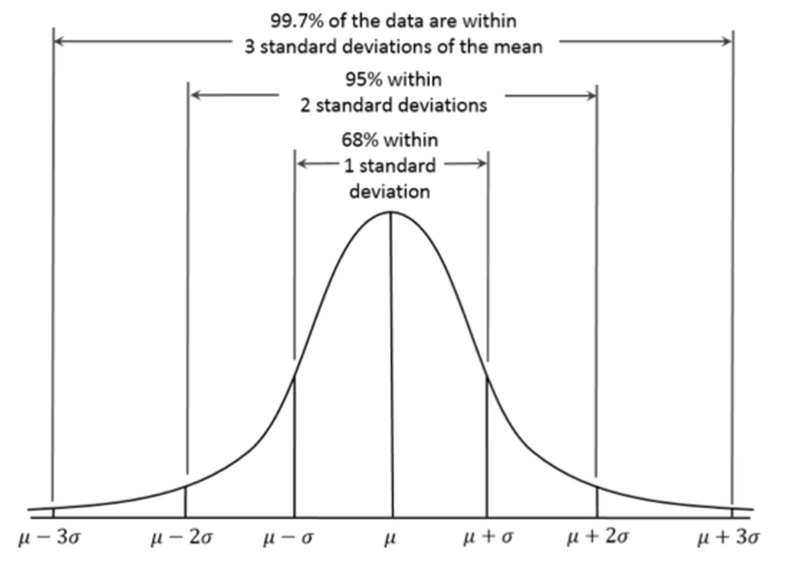# Statistical Infrequency Definition of Abnormality

Level:
AS, A Level
Board:
AQA, Edexcel, OCR, IB
Share:

This is a mathematical method for defining abnormality. This definition works on the idea that abnormality should be based on infrequency; if it occurs rarely then it is abnormal.

The mathematical element of the definition is about the idea that human attributes fall into a normal distribution within the population.

This means that there is a central average, or mean, and the rest of the population fall symmetrically above and below that mean. Standard deviation as a measure informs us how far scores fall on either side away from the mean.

In a characteristic or attribute that falls into a normal distribution, like IQ, 68% of the population fall within one standard deviation from the mean. 95% of the population fall within two standard deviations from the mean. As the mean of IQ is 100 and the standard deviation for IQ is 15 that means that 68% of the population will score between 85- 115 on an IQ test and 95% of the population will score between 70 and 130.

This definition states that the 5% of the population that fall more than two standard deviations from the mean are abnormal. So, in the IQ example people who score below 70 and above 130 are abnormal.

This is illustrated in Figure 1 below.Figure 1 An illustration of the normal distribution and percentages of the population to fall within the sections of that distribution

Strengths of the definition

Intuitive: The idea that abnormality is when something does not occur, as stated by this definition, often is an accepted definition for the word and makes sense.

Objective: The mathematical nature of this definition means that it is clear what is defined as abnormal and what is not. There is no opinion involved which means there is no bias.

A useful overview: This definition looks at the whole picture, taking all the population into account so can give a useful insight into the whole picture of a particular characteristic.

Weaknesses of the definition

Inflexible: There is a degree of inflexibility with this definition. It states that scores in the top and bottom 2.5% of the population are abnormal, but we know that there is some behaviour which is deemed abnormal by the diagnostic manuals for mental illness that would not fit within that definition. For example 3% of the population is thought to suffer from OCD but some of those individuals may not be seen as abnormal because they are within 2 standard deviations of the mean. This means that the definition and the current criteria for defining mental health issues are at odds with each other.

Abnormal behaviours can occur frequently: It is not the case that abnormal, as in unhelpful and painful, behaviours occur rarely. Depression is an example, where it is argued that 25% of the population will experience a mental health issue at some point in their lives.

Culture: There is no consideration of cultural differences at all as it argues that the population of the world are contained within the normal distribution. There are variances between cultures and the mathematical nature of this definition does not allow for this. This ultimately means that abnormality could be widespread in one culture because of its comparison with the rest of the world.

Share:

Boston House,
214 High Street,
Boston Spa,
West Yorkshire,